# 2.6 Electron-Phonon Interaction

The electron-phonon interaction HAMILTONian for CNTs can be written as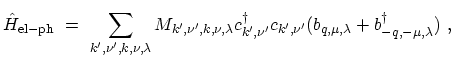(2.14)

where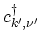and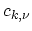are the electron creation and annihilation operators, respectively,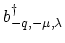and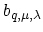are the phonon creation and annihilation operators, respectively, and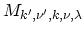is the electron-phonon matrix element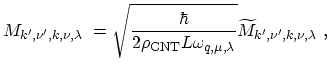(2.15)

where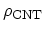is the mass density of the CNT,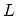is the normalization length, and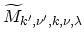is the reduced electron-phonon matrix element of the transition from the initial electronic state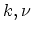to the final state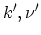, where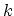is the wave-vector and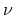is the azimuthal quantum number of electrons. Because of energy conservation for a scattering event it holds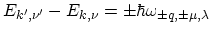, where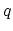is the wave-vector,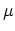is the azimuthal quantum number, and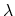is the polarization of the phonon. The matrix element obeys selection rules arising from wave-vector and azimuthal quantum number conservation,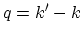and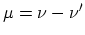.

Because in the CNT two degrees of freedom are confined, an electron can only be scattered forward or backward in the axial direction, preserving or changing the sign of the band-velocity, respectively. The scattering processes invoke either intra-subband or inter-subband transitions. The intra-subband processes are important for the electrical and the heat transport in CNTs and for the relaxation of an excited electron or hole in the same subband. The inter-subband processes contribute to the radiation-less relaxation of electrons (holes) from a given subband to a subband with a lower (higher) energy . The scattering of electrons can take place within a given valley or between two valleys. The two possibilities being termed intra-valley and inter-valley scattering processes.

Subsections

M. Pourfath: Numerical Study of Quantum Transport in Carbon Nanotube-Based Transistors